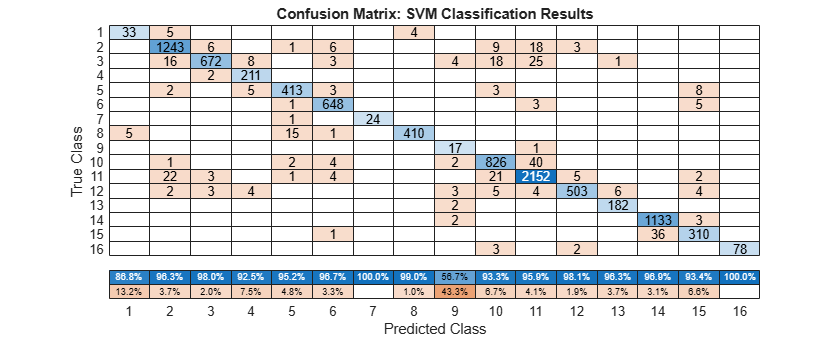# Classify Hyperspectral Image Using Support Vector Machine Classifier

This example shows how to preprocess a hyperspectral image and classify it using a support vector machine (SVM) classifier. To run this example, you must have a license for Statistics and Machine Learning Toolbox™.

Hyperspectral images are acquired over multiple spectral bands and consist of several hundred band images, each representing the same scene across different wavelengths. This example uses the Indian Pines data set, acquired by the Airborne Visible/Infrared Imaging Spectrometer (AVIRIS) sensor across a wavelength range of 400 to 2500 nm. This data set contains 16 classes and 220 band images. Each image is of size 145-by-145 pixels.In this example, you:

1. Preprocess a hyperspectral image using a 2-D Gaussian filter.

2. Perform classification using a SVM classifier.

3. Display classification results, such as the classification accuracy, classification map, and confusion matrix.

Read the hyperspectral data into the workspace by using the `hypercube` function.

`hcube = hypercube("indian_pines.dat");`

Load the ground truth for the data set into the workspace.

```gtLabel = load("indian_pines_gt.mat"); gtLabel = gtLabel.indian_pines_gt; numClasses = 16;```

### Preprocess Hyperspectral Data

Remove the water absorption bands from the data by using the `removeBands` function.

```band = [104:108 150:163 220]; % Water absorption bands newhcube = removeBands(hcube,BandNumber=band);```

Estimate RGB images of the input hypercube by using the `colorize` function.

`rgbImg = colorize(newhcube,method="rgb");`

Apply a Gaussian filter ($\sigma =2$) to each band image of the hyperspectral data using the `imgaussfilt` function, and then convert them to grayscale images.

```hsData = newhcube.DataCube; [M,N,C] = size(hsData); hsDataFiltered = zeros(size(hsData)); for band = 1:C bandImage = hsData(:,:,band); bandImageFiltered = imgaussfilt(bandImage,2); bandImageGray = mat2gray(bandImageFiltered); hsDataFiltered(:,:,band) = uint8(bandImageGray*255); end```

### Prepare Data for Classification

Reshape the filtered hyperspectral data to a set of feature vectors containing filtered spectral responses for each pixel.

`DataVector = reshape(hsDataFiltered,[M*N C]);`

Reshape the ground truth image to a vector containing class labels.

`gtVector = gtLabel(:);`

Find the location indices of the ground truth vector that contain class labels. Discard labels with the value `0`, as they are unlabeled and do not represent a class.

```gtLocs = find(gtVector~=0); classLabel = gtVector(gtLocs);```

Create training and testing location indices for the specified training percentage by using the `cvpartition` (Statistics and Machine Learning Toolbox) function.

```per = 0.1; % Training percentage cv = cvpartition(classLabel,HoldOut=1-per);```

Split the ground truth location indices into training and testing location indices.

```locTrain = gtLocs(cv.training); locTest = gtLocs(~cv.training);```

### Classify Using SVM

Train the SVM classifier using the `fitcecoc` (Statistics and Machine Learning Toolbox) function.

`svmMdl = fitcecoc(DataVector(locTrain,:),gtVector(locTrain,:));`

Test the SVM classifier using the test data.

`[svmLabelOut,~] = predict(svmMdl,DataVector(locTest,:));`

### Display Classification Results

Calculate and display the classification accuracy.

```svmAccuracy = sum(svmLabelOut == gtVector(locTest))/numel(locTest); disp(["Overall Accuracy (OA) of the test data using SVM = ",num2str(svmAccuracy)])```
``` "Overall Accuracy (OA) of the t..." "0.96" ```

Create an SVM classification map.

```svmPredLabel = gtLabel; svmPredLabel(locTest) = svmLabelOut;```

Display the RGB image, ground truth map, and SVM classification map.

```cmap = parula(numClasses); figure tiledlayout(1,3,TileSpacing="loose") nexttile imshow(rgbImg) title("RGB Image") nexttile imshow(gtLabel,cmap) title("Ground Truth Map") nexttile imshow(svmPredLabel,cmap) colorbar title("SVM Classification Map")```Display the confusion matrix.

```fig = figure; confusionchart(gtVector(locTest),svmLabelOut,ColumnSummary="column-normalized") fig_Position = fig.Position; fig_Position(3) = fig_Position(3)*1.5; fig.Position = fig_Position; title("Confusion Matrix: SVM Classification Results")```Patro, Ram Narayan, Subhashree Subudhi, Pradyut Kumar Biswal, and Fabio Dell’acqua. “A Review of Unsupervised Band Selection Techniques: Land Cover Classification for Hyperspectral Earth Observation Data.” IEEE Geoscience and Remote Sensing Magazine 9, no. 3 (September 2021): 72–111. https://doi.org/10.1109/MGRS.2021.3051979.